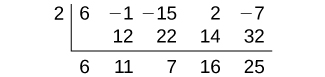# 5.5 Zeros of polynomial functions

 Page 1 / 14
In this section, you will:
• Evaluate a polynomial using the Remainder Theorem.
• Use the Factor Theorem to solve a polynomial equation.
• Use the Rational Zero Theorem to find rational zeros.
• Find zeros of a polynomial function.
• Use the Linear Factorization Theorem to find polynomials with given zeros.
• Use Descartes’ Rule of Signs.
• Solve real-world applications of polynomial equations

A new bakery offers decorated sheet cakes for children’s birthday parties and other special occasions. The bakery wants the volume of a small cake to be 351 cubic inches. The cake is in the shape of a rectangular solid. They want the length of the cake to be four inches longer than the width of the cake and the height of the cake to be one-third of the width. What should the dimensions of the cake pan be?

This problem can be solved by writing a cubic function and solving a cubic equation for the volume of the cake. In this section, we will discuss a variety of tools for writing polynomial functions and solving polynomial equations.

## Evaluating a polynomial using the remainder theorem

In the last section, we learned how to divide polynomials. We can now use polynomial division to evaluate polynomials using the Remainder Theorem    . If the polynomial is divided by $\text{\hspace{0.17em}}x–k,\text{\hspace{0.17em}}$ the remainder may be found quickly by evaluating the polynomial function at $\text{\hspace{0.17em}}k,\text{\hspace{0.17em}}$ that is, $\text{\hspace{0.17em}}f\left(k\right)\text{\hspace{0.17em}}$ Let’s walk through the proof of the theorem.

Recall that the Division Algorithm    states that, given a polynomial dividend $\text{\hspace{0.17em}}f\left(x\right)\text{\hspace{0.17em}}$ and a non-zero polynomial divisor $\text{\hspace{0.17em}}d\left(x\right)\text{\hspace{0.17em}}$ where the degree of $\text{\hspace{0.17em}}\text{\hspace{0.17em}}d\left(x\right)\text{\hspace{0.17em}}$ is less than or equal to the degree of $\text{\hspace{0.17em}}f\left(x\right)$ , there exist unique polynomials $\text{\hspace{0.17em}}q\left(x\right)\text{\hspace{0.17em}}$ and $\text{\hspace{0.17em}}r\left(x\right)\text{\hspace{0.17em}}$ such that

$\text{\hspace{0.17em}}f\left(x\right)=d\left(x\right)q\left(x\right)+r\left(x\right)$

If the divisor, $\text{\hspace{0.17em}}d\left(x\right),\text{\hspace{0.17em}}$ is $\text{\hspace{0.17em}}x-k,\text{\hspace{0.17em}}$ this takes the form

$f\left(x\right)=\left(x-k\right)q\left(x\right)+r$

Since the divisor $\text{\hspace{0.17em}}x-k\text{\hspace{0.17em}}$ is linear, the remainder will be a constant, $\text{\hspace{0.17em}}r.\text{\hspace{0.17em}}$ And, if we evaluate this for $\text{\hspace{0.17em}}x=k,\text{\hspace{0.17em}}$ we have

$\begin{array}{ccc}\hfill f\left(k\right)& =& \left(k-k\right)q\left(k\right)+r\hfill \\ & =& 0\cdot q\left(k\right)+r\hfill \\ & =& r\hfill \end{array}$

In other words, $\text{\hspace{0.17em}}f\left(k\right)\text{\hspace{0.17em}}$ is the remainder obtained by dividing $\text{\hspace{0.17em}}f\left(x\right)\text{\hspace{0.17em}}$ by $\text{\hspace{0.17em}}x-k.\text{\hspace{0.17em}}$

## The remainder theorem

If a polynomial $\text{\hspace{0.17em}}f\left(x\right)\text{\hspace{0.17em}}$ is divided by $\text{\hspace{0.17em}}x-k,\text{\hspace{0.17em}}$ then the remainder is the value $\text{\hspace{0.17em}}f\left(k\right).\text{\hspace{0.17em}}$

Given a polynomial function $\text{\hspace{0.17em}}f,$ evaluate $\text{\hspace{0.17em}}f\left(x\right)\text{\hspace{0.17em}}$ at $\text{\hspace{0.17em}}x=k\text{\hspace{0.17em}}$ using the Remainder Theorem.

1. Use synthetic division to divide the polynomial by $\text{\hspace{0.17em}}x-k.\text{\hspace{0.17em}}$
2. The remainder is the value $\text{\hspace{0.17em}}f\left(k\right).\text{\hspace{0.17em}}$

## Using the remainder theorem to evaluate a polynomial

Use the Remainder Theorem to evaluate $\text{\hspace{0.17em}}f\left(x\right)=6{x}^{4}-{x}^{3}-15{x}^{2}+2x-7\text{\hspace{0.17em}}$ at $\text{\hspace{0.17em}}x=2.\text{\hspace{0.17em}}$

To find the remainder using the Remainder Theorem, use synthetic division to divide the polynomial by $\text{\hspace{0.17em}}x-2.\text{\hspace{0.17em}}$The remainder is 25. Therefore, $\text{\hspace{0.17em}}f\left(2\right)=25.\text{\hspace{0.17em}}$

Use the Remainder Theorem to evaluate $\text{\hspace{0.17em}}f\left(x\right)=2{x}^{5}-3{x}^{4}-9{x}^{3}+8{x}^{2}+2\text{\hspace{0.17em}}$ at $\text{\hspace{0.17em}}x=-3.\text{\hspace{0.17em}}$

$\text{\hspace{0.17em}}f\left(-3\right)=-412\text{\hspace{0.17em}}$

## Using the factor theorem to solve a polynomial equation

The Factor Theorem is another theorem that helps us analyze polynomial equations. It tells us how the zeros of a polynomial are related to the factors. Recall that the Division Algorithm.

$f\left(x\right)=\left(x-k\right)q\left(x\right)+r$

If $\text{\hspace{0.17em}}k\text{\hspace{0.17em}}$ is a zero, then the remainder $\text{\hspace{0.17em}}r\text{\hspace{0.17em}}$ is $\text{\hspace{0.17em}}f\left(k\right)=0\text{\hspace{0.17em}}$ and $\text{\hspace{0.17em}}f\left(x\right)=\left(x-k\right)q\left(x\right)+0\text{\hspace{0.17em}}$ or $\text{\hspace{0.17em}}f\left(x\right)=\left(x-k\right)q\left(x\right).\text{\hspace{0.17em}}$

Notice, written in this form, $\text{\hspace{0.17em}}x-k\text{\hspace{0.17em}}$ is a factor of $\text{\hspace{0.17em}}f\left(x\right).\text{\hspace{0.17em}}$ We can conclude if $\text{\hspace{0.17em}}k\text{\hspace{0.17em}}$ is a zero of $\text{\hspace{0.17em}}f\left(x\right),\text{\hspace{0.17em}}$ then $\text{\hspace{0.17em}}x-k\text{\hspace{0.17em}}$ is a factor of $f\left(x\right).\text{\hspace{0.17em}}$

#### Questions & Answers

if sin15°=√p, express the following in terms of p
Norman Reply
prove sin²x+cos²x=3+cos4x
Kiddy Reply
the difference between two signed numbers is -8.if the minued is 5,what is the subtrahend
jeramie Reply
the difference between two signed numbers is -8.if the minuend is 5.what is the subtrahend
jeramie
what are odd numbers
micheal Reply
numbers that leave a remainder when divided by 2
Thorben
1,3,5,7,... 99,...867
Thorben
7%2=1, 679%2=1, 866245%2=1
Thorben
the third and the seventh terms of a G.P are 81 and 16, find the first and fifth terms.
Suleiman Reply
if a=3, b =4 and c=5 find the six trigonometric value sin
Martin Reply
ask
Ans
pls how do I factorize x⁴+x³-7x²-x+6=0
Gift Reply
in a function the input value is called
Rimsha Reply
how do I test for values on the number line
Modesta Reply
if a=4 b=4 then a+b=
Rimsha Reply
a+b+2ab
Kin
commulative principle
DIOSDADO
a+b= 4+4=8
Mimi
If a=4 and b=4 then we add the value of a and b i.e a+b=4+4=8.
Tariq
what are examples of natural number
sani Reply
an equation for the line that goes through the point (-1,12) and has a slope of 2,3
Katheryn Reply
3y=-9x+25
Ishaq
show that the set of natural numberdoes not from agroup with addition or multiplication butit forms aseni group with respect toaaddition as well as multiplication
Komal Reply
x^20+x^15+x^10+x^5/x^2+1
Urmila Reply
evaluate each algebraic expression. 2x+×_2 if ×=5
Sarch Reply

### Read also:

#### Get Jobilize Job Search Mobile App in your pocket Now!

Source:  OpenStax, Algebra and trigonometry. OpenStax CNX. Nov 14, 2016 Download for free at https://legacy.cnx.org/content/col11758/1.6
Google Play and the Google Play logo are trademarks of Google Inc.

Notification Switch

Would you like to follow the 'Algebra and trigonometry' conversation and receive update notifications?ByByBy OpenStaxByBy OpenStaxBy Madison ChristianBy Jams KaloBy Keyaira BraxtonBy Rohini AjayBy Yasser IbrahimBy OpenStaxBy OpenStax